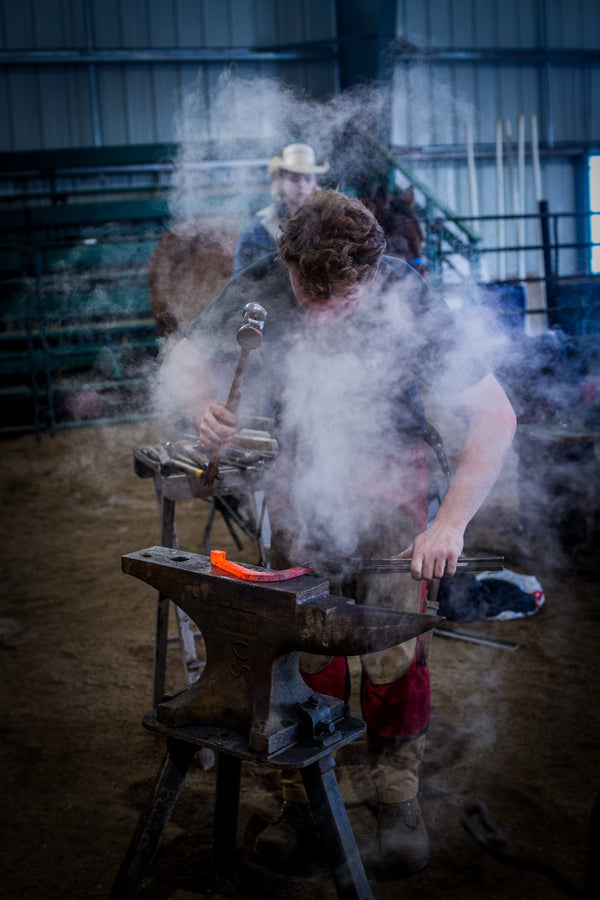0

# Gauging Drill Bit for Gas Orifices

Exclusive Pressure-bonded high-speed steel gauging drills. These small diameter precision drills are great for sizing gas orifices, burners, and carburetor jets. High-Speed Gauging drill sold without hex handles to enable you to use the TL072 pin vise or small electric drill.

Sizes Available

• # 50 = 0.07 Decimal Inches
• # 51 = 0.067 Decimal Inches
• # 52 = 0.0635 Decimal Inches
• # 57 = 0.043 Decimal Inches
• # 58 = 0.042 Decimal Inches
• # 59 = 0.041 Decimal Inches
• # 61 = 0.039 Decimal Inches
• # 62 = 0.038 Decimal Inches
• # 64 = 0.036 Decimal Inches
• # 66 = 0.033 Decimal Inches
• # 69 = 0.0292 Decimal Inches
• # 71 = 0.026 Decimal Inches

## Subscribe